# 量化分析数字货币市场

Author: , Created: 2019-08-16 10:37:23, Updated: 2019-08-19 14:19:11

# 一种数据驱动的数字货币投机分析方法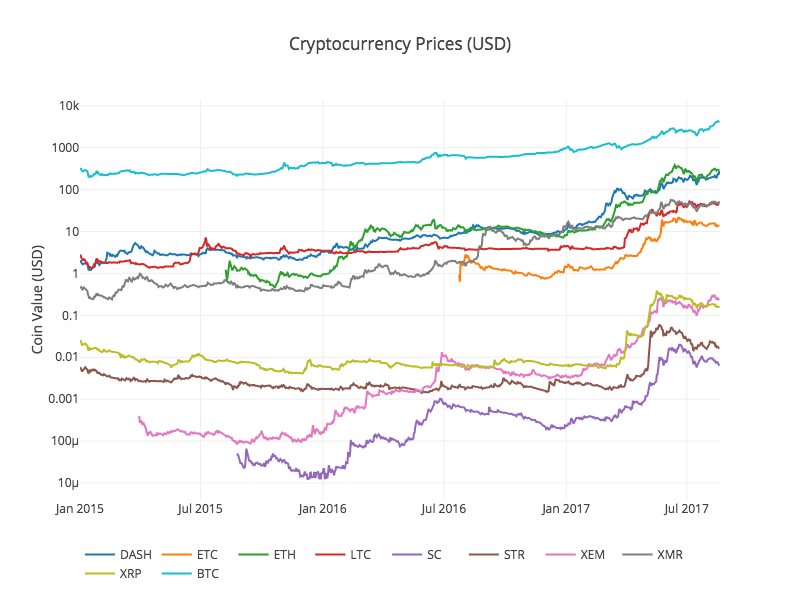# 第一步：搭建我们的数据工作环境

• 发明者量化的托管者系统

## 1.2 创建一个Anaconda的数据分析项目环境

Anaconda安装完成后，我们需要创建一个新的环境来管理我们的依赖包。在linux命令行界面，我们输入：

``````conda create --name cryptocurrency-analysis python=3
``````

``````source activate cryptocurrency-analysis (linux/MacOS操作)

activate cryptocurrency-analysis (windows操作系统)
``````

``````conda install numpy pandas nb_conda jupyter plotly
``````

## 1.3 创建一个Jupyter Notebook

``````jupyter notebook
``````

``````Python [conda env:cryptocurrency-analysis]
``````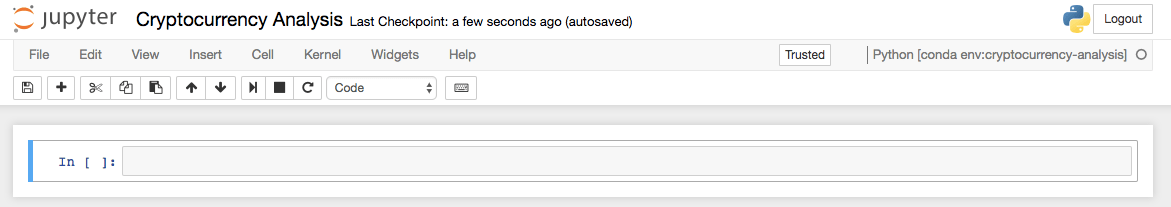## 1.4 导入依赖包

``````import os
import numpy as np
import pandas as pd
import pickle
from datetime import datetime
``````

``````import plotly.offline as py
import plotly.graph_objs as go
import plotly.figure_factory as ff
py.init_notebook_mode(connected=True)
``````

# 第二步：获取数字货币的价格信息

## 2.1 编写Quandl搜集数据函数

``````def get_quandl_data(quandl_id):
# 下载和缓冲来自Quandl的数据列
cache_path = '{}.pkl'.format(quandl_id).replace('/','-')
try:
f = open(cache_path, 'rb')
except (OSError, IOError) as e:
df = quandl.get(quandl_id, returns="pandas")
df.to_pickle(cache_path)
print('Cached {} at {}'.format(quandl_id, cache_path))
return df
``````

## 2.2 获取Kraken交易所的数字货币价格数据

``````# 获取Kraken比特币交易所的价格
btc_usd_price_kraken = get_quandl_data('BCHARTS/KRAKENUSD')
``````

``````btc_usd_price_kraken.head()
``````

BTC Open High Low Close Volume (BTC) Volume (Currency) Weighted Price
2014-01-07 874.67040 892.06753 810.00000 810.00000 15.622378 13151.472844 841.835522
2014-01-08 810.00000 899.84281 788.00000 824.98287 19.182756 16097.329584 839.156269
2014-01-09 825.56345 870.00000 807.42084 841.86934 8.158335 6784.249982 831.572913
2014-01-10 839.99000 857.34056 817.00000 857.33056 8.024510 6780.220188 844.938794
2014-01-11 858.20000 918.05471 857.16554 899.84105 18.748285 16698.566929 890.671709

``````# 做出BTC价格的表格
btc_trace = go.Scatter(x=btc_usd_price_kraken.index, y=btc_usd_price_kraken['Weighted Price'])
py.iplot([btc_trace])
``````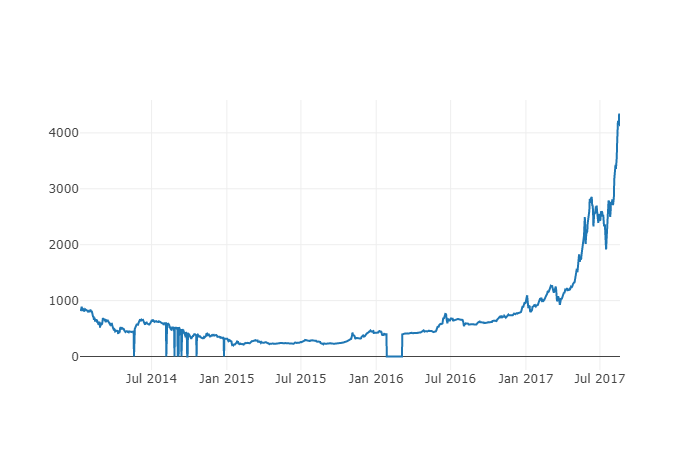## 2.3 从主流的比特币交易所获取价格数据

``````# 下载COINBASE，BITSTAMP和ITBIT的价格数据
exchanges = ['COINBASE','BITSTAMP','ITBIT']

exchange_data = {}

exchange_data['KRAKEN'] = btc_usd_price_kraken

for exchange in exchanges:
exchange_code = 'BCHARTS/{}USD'.format(exchange)
btc_exchange_df = get_quandl_data(exchange_code)
exchange_data[exchange] = btc_exchange_df
``````

## 2.4 将所有数据整合到一个数据帧之中

``````def merge_dfs_on_column(dataframes, labels, col):
'''Merge a single column of each dataframe into a new combined dataframe'''
series_dict = {}
for index in range(len(dataframes)):
series_dict[labels[index]] = dataframes[index][col]

return pd.DataFrame(series_dict)
``````

``````# 整合所有数据帧
btc_usd_datasets = merge_dfs_on_column(list(exchange_data.values()), list(exchange_data.keys()), 'Weighted Price')
``````

``````btc_usd_datasets.tail()
``````

BTC BITSTAMP COINBASE ITBIT KRAKEN
2017-08-14 4210.154943 4213.332106 4207.366696 4213.257519
2017-08-15 4101.447155 4131.606897 4127.036871 4149.146996
2017-08-16 4193.426713 4193.469553 4190.104520 4187.399662
2017-08-17 4338.694675 4334.115210 4334.449440 4346.508031
2017-08-18 4182.166174 4169.555948 4175.440768 4198.277722

## 2.5 价格数据的可视化过程

``````def df_scatter(df, title, seperate_y_axis=False, y_axis_label='', scale='linear', initial_hide=False):
'''Generate a scatter plot of the entire dataframe'''
label_arr = list(df)
series_arr = list(map(lambda col: df[col], label_arr))

layout = go.Layout(
title=title,
legend=dict(orientation="h"),
xaxis=dict(type='date'),
yaxis=dict(
title=y_axis_label,
showticklabels= not seperate_y_axis,
type=scale
)
)

y_axis_config = dict(
overlaying='y',
showticklabels=False,
type=scale )

visibility = 'visible'
if initial_hide:
visibility = 'legendonly'

# 每个系列的表格跟踪
trace_arr = []
for index, series in enumerate(series_arr):
trace = go.Scatter(
x=series.index,
y=series,
name=label_arr[index],
visible=visibility
)

# 为系列添加单独的轴
if seperate_y_axis:
trace['yaxis'] = 'y{}'.format(index + 1)
layout['yaxis{}'.format(index + 1)] = y_axis_config
trace_arr.append(trace)

fig = go.Figure(data=trace_arr, layout=layout)
py.iplot(fig)
``````

``````# 绘制所有BTC交易价格
df_scatter(btc_usd_datasets, 'Bitcoin Price (USD) By Exchange')
``````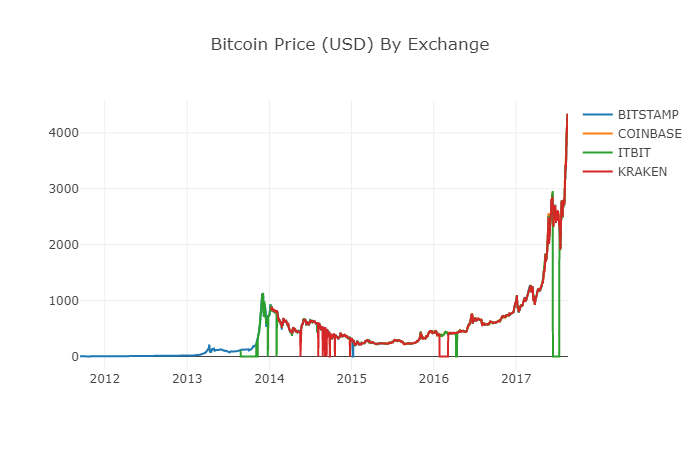## 2.6 清理并加总价格数据

``````# 清除"0"值
btc_usd_datasets.replace(0, np.nan, inplace=True)
``````

``````# 绘制修订后的数据框
df_scatter(btc_usd_datasets, 'Bitcoin Price (USD) By Exchange')
``````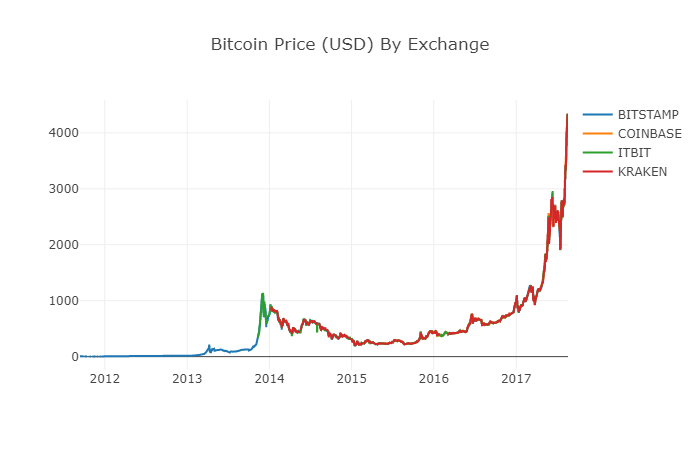``````# 将平均BTC价格计算为新列
btc_usd_datasets['avg_btc_price_usd'] = btc_usd_datasets.mean(axis=1)
``````

``````# 绘制平均BTC价格
btc_trace = go.Scatter(x=btc_usd_datasets.index, y=btc_usd_datasets['avg_btc_price_usd'])
py.iplot([btc_trace])
``````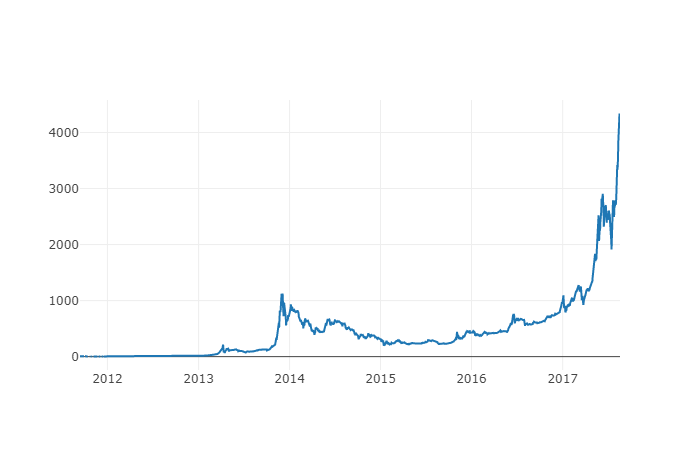# 第三步：收集山寨币（Altcoins）的价格

## 3.1 通过Poloniex交易所的API定义辅助函数

``````def get_json_data(json_url, cache_path):
try:
f = open(cache_path, 'rb')
except (OSError, IOError) as e:
df.to_pickle(cache_path)
print('Cached {} at {}'.format(json_url, cache_path))
return df
``````

``````base_polo_url = 'https://poloniex.com/public?command=returnChartData&currencyPair={}&start={}&end={}&period={}'
start_date = datetime.strptime('2015-01-01', '%Y-%m-%d') # 从2015年开始获取数据
end_date = datetime.now() # 直到今天
pediod = 86400 # pull daily data (86,400 seconds per day)

def get_crypto_data(poloniex_pair):
'''Retrieve cryptocurrency data from poloniex'''
json_url = base_polo_url.format(poloniex_pair, start_date.timestamp(), end_date.timestamp(), pediod)
data_df = get_json_data(json_url, poloniex_pair)
data_df = data_df.set_index('date')
return data_df
``````

## 3.2 从Poloniex下载交易价格数据

``````altcoins = ['ETH','LTC','XRP','ETC','STR','DASH','SC','XMR','XEM']

altcoin_data = {}
for altcoin in altcoins:
coinpair = 'BTC_{}'.format(altcoin)
crypto_price_df = get_crypto_data(coinpair)
altcoin_data[altcoin] = crypto_price_df
``````

``````altcoin_data['ETH'].tail()
``````
ETH Open High Low Close Volume (BTC) Volume (Currency) Weighted Price
2017-08-18 0.070510 0.071000 0.070170 0.070887 17364.271529 1224.762684 0.070533
2017-08-18 0.071595 0.072096 0.070004 0.070510 26644.018123 1893.136154 0.071053
2017-08-18 0.071321 0.072906 0.070482 0.071600 39655.127825 2841.549065 0.071657
2017-08-19 0.071447 0.071855 0.070868 0.071321 16116.922869 1150.361419 0.071376
2017-08-19 0.072323 0.072550 0.071292 0.071447 14425.571894 1039.596030 0.072066

## 3.3 把所有价格数据的货币单位统一为美元

``````# 将USD Price计算为每个altcoin数据帧中的新列
for altcoin in altcoin_data.keys():
altcoin_data[altcoin]['price_usd'] =  altcoin_data[altcoin]['weightedAverage'] * btc_usd_datasets['avg_btc_price_usd']
``````

``````# 将每个山寨币的美元价格合并为单个数据帧
combined_df = merge_dfs_on_column(list(altcoin_data.values()), list(altcoin_data.keys()), 'price_usd')
``````

``````# 将BTC价格添加到数据帧
combined_df['BTC'] = btc_usd_datasets['avg_btc_price_usd']
``````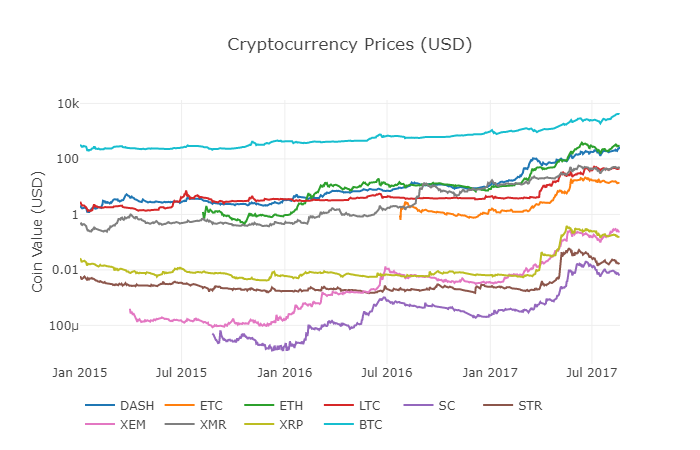## 3.4 开始进行相关性分析

2017.8.22修订说明:这部分的修改是为了在计算相关系数时使用每日回报率而不是价格的绝对值。

``````# 计算2016年数字货币的皮尔森相关系数
combined_df_2016 = combined_df[combined_df.index.year == 2016]
combined_df_2016.pct_change().corr(method='pearson')
``````
Name DASH ETC ETH LTC SC STR XEM XMR XRP BTC
DASH 1.000000 0.003992 0.122695 -0.012194 0.026602 0.058083 0.014571 0.121537 0.088657 -0.014040
ETC 0.003992 1.000000 -0.181991 -0.131079 -0.008066 -0.102654 -0.080938 -0.105898 -0.054095 -0.170538
ETH 0.122695 -0.181991 1.000000 -0.064652 0.169642 0.035093 0.043205 0.087216 0.085630 -0.006502
LTC -0.012194 -0.131079 -0.064652 1.000000 0.012253 0.113523 0.160667 0.129475 0.053712 0.750174
SC 0.026602 -0.008066 0.169642 0.012253 1.000000 0.143252 0.106153 0.047910 0.021098 0.035116
STR 0.058083 -0.102654 0.035093 0.113523 0.143252 1.000000 0.225132 0.027998 0.320116 0.079075
XEM 0.014571 -0.080938 0.043205 0.160667 0.106153 0.225132 1.000000 0.016438 0.101326 0.227674
XMR 0.121537 -0.105898 0.087216 0.129475 0.047910 0.027998 0.016438 1.000000 0.027649 0.127520
XRP 0.088657 -0.054095 0.085630 0.053712 0.021098 0.320116 0.101326 0.027649 1.000000 0.044161
BTC -0.014040 -0.170538 -0.006502 0.750174 0.035116 0.079075 0.227674 0.127520 0.044161 1.000000

``````def correlation_heatmap(df, title, absolute_bounds=True):
'''Plot a correlation heatmap for the entire dataframe'''
heatmap = go.Heatmap(
z=df.corr(method='pearson').as_matrix(),
x=df.columns,
y=df.columns,
colorbar=dict(title='Pearson Coefficient'),
)

layout = go.Layout(title=title)

if absolute_bounds:
heatmap['zmax'] = 1.0
heatmap['zmin'] = -1.0

fig = go.Figure(data=[heatmap], layout=layout)
py.iplot(fig)
``````
``````correlation_heatmap(combined_df_2016.pct_change(), "Cryptocurrency Correlations in 2016")
``````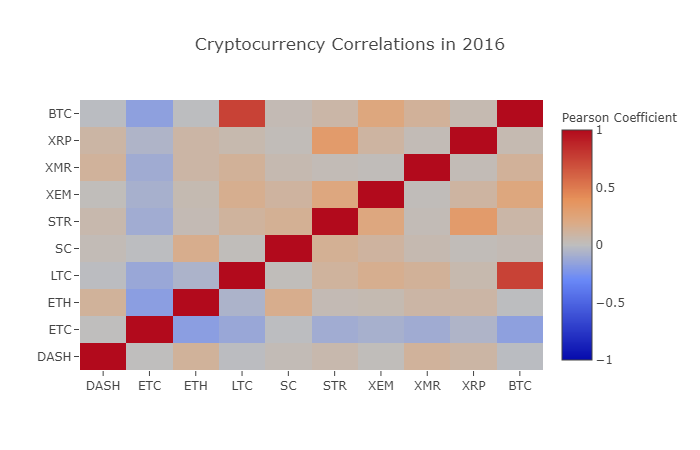``````combined_df_2017 = combined_df[combined_df.index.year == 2017]
combined_df_2017.pct_change().corr(method='pearson')
``````
Name DASH ETC ETH LTC SC STR XEM XMR XRP BTC
DASH 1.000000 0.384109 0.480453 0.259616 0.191801 0.159330 0.299948 0.503832 0.066408 0.357970
ETC 0.384109 1.000000 0.602151 0.420945 0.255343 0.146065 0.303492 0.465322 0.053955 0.469618
ETH 0.480453 0.602151 1.000000 0.286121 0.323716 0.228648 0.343530 0.604572 0.120227 0.421786
LTC 0.259616 0.420945 0.286121 1.000000 0.296244 0.333143 0.250566 0.439261 0.321340 0.352713
SC 0.191801 0.255343 0.323716 0.296244 1.000000 0.417106 0.287986 0.374707 0.248389 0.377045
STR 0.159330 0.146065 0.228648 0.333143 0.417106 1.000000 0.396520 0.341805 0.621547 0.178706
XEM 0.299948 0.303492 0.343530 0.250566 0.287986 0.396520 1.000000 0.397130 0.270390 0.366707
XMR 0.503832 0.465322 0.604572 0.439261 0.374707 0.341805 0.397130 1.000000 0.213608 0.510163
XRP 0.066408 0.053955 0.120227 0.321340 0.248389 0.621547 0.270390 0.213608 1.000000 0.170070
BTC 0.357970 0.469618 0.421786 0.352713 0.377045 0.178706 0.366707 0.510163 0.170070 1.000000

``````correlation_heatmap(combined_df_2017.pct_change(), "Cryptocurrency Correlations in 2017")
``````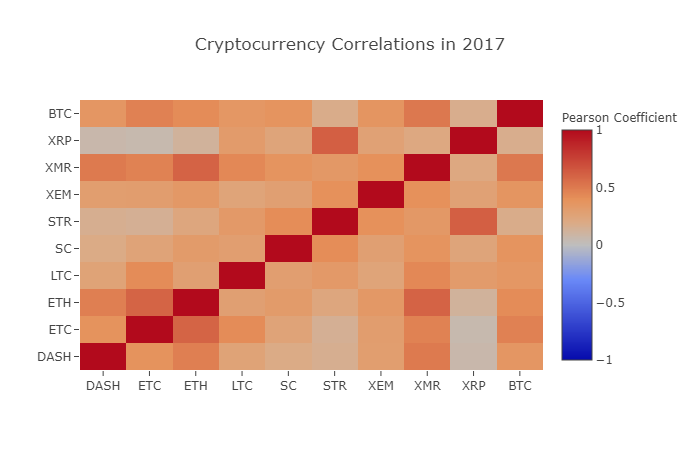# 好了，轮到你了！

• 为整个分析添加更多数字货币的数据
• 调整相关性分析的时间范围和颗粒度，以得到优化的或粗粒度的趋势视图。
• 从交易量或区块链数据挖掘集中寻找趋势。相较于原始的价格数据，如果你想预测未来价格波动，你可能更需要买/卖量的比率数据。
• 在股票、商品、法定货币上加入价格数据来决定他们当中哪一项与数字货币具有相关性（但是，别忘了那句老话“相关不蕴含因果”）